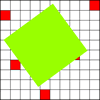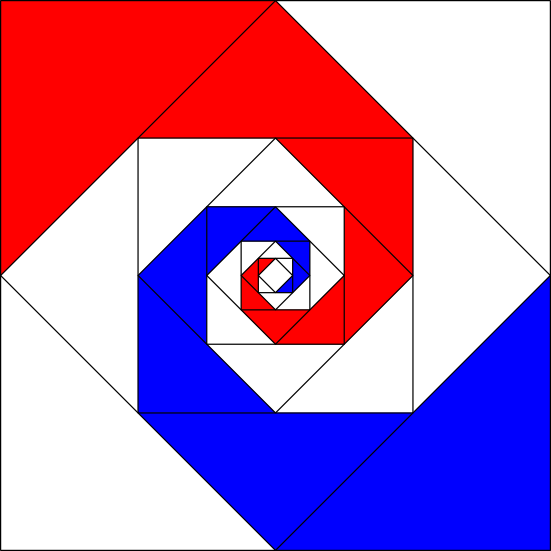You may also likeWhich of these roads will satisfy a Munchkin builder?How Random!

Explore this interactivity and see if you can work out what it does. Could you use it to estimate the area of a shape?For Richer for Poorer

Charlie has moved between countries and the average income of both has increased. How can this be so?

Baravelle

Baravelle

Look at this image for a short while before turning away.
Can you:

• Recreate the image?
• Describe the image?
• Say some mathematical things about what you notice?
• Think of some mathematical questions you would like to ask about it?

Here is a pdf document containing the image.This image is taken from the NRICH Mathematics Posters CD called "Exploring Squares" published by Virtual Image. More details of this and the "Exploring Circles" CD can be foundhere.

Why do this problem

The aim of using this image is to encourage discussion about the different ways of seeing, and to pose questions that can form the focus of further investigation.

Possible approach

You can find a pdf with the image here. Look at the image for about a minute and then "hide it". Then share ideas.

Follow up by selecting one or two of the ideas for the group to explore further, for example:
• Recreating the image
• Finding what fraction of the whole the areas is shaded red, blue and white.

Key questions

Can you continue the pattern inwards? Outwards?
Can you give a convincing argument for the fractions of the whole that you can see?

Possible Extension

Consider summing infinite series.
What are the ratios of the lengths of the sides of the triangles?

Possible support

Can you find the fractions and or recreate the shape by folding? Some learners might like to try the problem Inside Seven Squares.